Maths-
General
Easy

Question

# Find the :a. Area of triangle whose three sides are 8 cm, 15 cm, 17 cm longb .Find the altitude from opposite vertex to the side whose length is 15cmHint:

## The correct answer is: a) 60 cm2 ,b) 8 cm

### It is given that a = 8 , b = 15 , c = 17(a) Using heron’s formulaArea of triangle =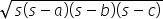where s =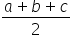s =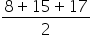= 20Area of triangle =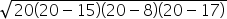=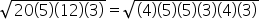= (4)(5)(3) = 60 cm2(b) It is given that base, b = 15 cmLet height be hArea of triangle =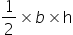60  =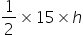8 = hSo, height of the triangle = 8 cmNOTE – We can prove that given triangle is a right-angled triangle using Pythagoras theorem.#### With Turito Foundation.#### Get an Expert Advice From Turito.